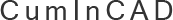CumInCAD is a Cumulative Index about publications in Computer Aided Architectural Design supported by the sibling associations ACADIA, CAADRIA, eCAADe, SIGraDi, ASCAAD and CAAD futures
 PDF papersReferences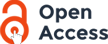authors Owen, J.C. 1991 Algebraic Solution for Geometry from Dimensional Constraints ACM Symp. Found. of Solid Modeling, Austin TX, pp. 397-407 We investigate general configurations of distance and angle dimensions between points, lines and circles on a plane. A simple graphical representation is described for the system of coupled ctuadratic equations which results from treating the geometries as variables and the dimensions as defining equations. For many configurations of practical interest we show that these equations are poorly suited to numerical solution. We describe an algorithm for computing the solution to a subset of all possible configurations of geometry and dimensions using purely algebraic methods (in fact the usual arithmetic operations plus square roots). We show that this algorithm solves for all configurations of a practically useful type and that it solves for any configuration which can in principle be solved using these algebraic operations. Specifically, we use the Galois theory of equations to show that the following statements are equivalent. 1. The geometry can be constructed in principle on a drawing board using a ruler and compasses. 2. The coordinates of the geometries can be computed algebraically using only arithmetic operations plus square root. 3. The coordinates of the geometries lie in a normal field extension over the dimension values of degree 2n for some n. 4. For general (i.e. algebraically independent) dimension values the algorithm described will compute the geometries. We also describe a working implementation of the algorithm and describe some extensions to the basic ideaa which are necessary to make it a practically useful way to specify geometry by means of dimensional constraints. other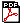file.pdf (817,528 bytes) Content-type: text/plain 2003/04/23 13:50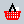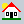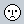CUMINCAD Papers